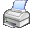﻿ bCell:Compare()

# bCell:Equal()Purpose

Compares the column and row of this cell with the passed cell.

Class

bCell

Type

Method

Syntax

<oCell>:Compare(

<oCell>

<iOptions>

) Æ iResult

Arguments

 The cell, whose values should to be compared.
 Data Type: bCell

 Options which should to be considered. The options below are supported:

 Option Description BCO_STARTCELL The cells are compared as a start cell.   Notes: If the value of access bCell:ColumnPercentage or bCell:RowPercentage is BCELL_PERCENTAGE_UNDEFINED, then the value BCELL_PERCENTAGE_NULL is used instead. BCO_ENDCELL The cells are compared as a end cell.   Notes: If the value of access bCell:ColumnPercentage or bCell:RowPercentage is BCELL_PERCENTAGE_UNDEFINED, then the value BCELL_PERCENTAGE_MAX is used instead.

 Data Type: DWord

Return Value

 iResult A numerical value that defines whether the passed cell is equal or before or behind this cell. If both cells are equal, then the value BCCR_EQUAL will be returned. Otherwise the LO-Word contains the result for the column value and the HI-Word the result for the row value. The following values may be returned:

 Value Description BCCR_BEFORE The column value or row value of the passed cell is before this cell. BCCR_EQUAL The column value or row value of the passed cell is equal. BCCR_BEHIND The column value or row value of the passed cell is behind this cell.

 Data Type: DWord

Description

bCell:Compare() compares the column values or the row values of both cells.

bCell:Column

bCell:ColumnPercentage

bCell:GetColumnValue()

bCell:GetRowValue()

bCell:Row

bCell:RowPercentage